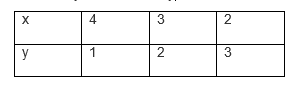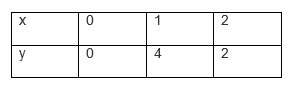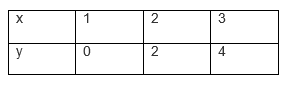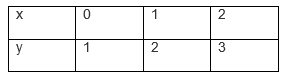## Ex 3.2 Question 1.

1. Form the pair of linear equations in the following problems, and find their solutions graphically.

(i) 10 students of Class X took part in a Mathematics quiz. If the number of girls is 4 more than the number of boys, find the number of boys and girls who took part in the quiz.

(ii) 5 pencils and 7 pens together cost 50, whereas 7 pencils and 5 pens together cost 46. Find the cost of one pencil and that of one pen.

Solution:

(i) Let the number of girls = x  and the number of boys in the class = y respectively.
x + y = 10
x – y = 4
x + y = 10x = 10 – y
Three solutions of this equation are

 x 5 4 6 y 5 6 4

x – y = 4x = 4 + y
Three solutions of this equation are

 x 5 4 3 y 1 0 -1Two lines cross each other at the point (7, 3).
∴There are 7 girls and 3 boys in the class. So, x = 7 and y = 3.

(ii) Let the cost of one pencil  = ₹ X and one pen = ₹ y respectively.

5x + 7y = 50
7x + 5y = 46
5x + 7y = 50 or  x = (50-7y)/5
Three solutions of this equation are

 x 3 10 -4 y 5 0 10

7x + 5y = 46 or x = (46-5y)/7
Three solutions of this equation are

 x 8 3 -2 y -2 5 12Two lines cross each other at the point (3, 5).
∴The cost of a pencil is 3/- and the cost of a pen is 5/- So, x = 3 and y = 5.

## Ex 3.2 Question 2.

On comparing the ratios a1/a2,b1/b1,c1/c2 find out whether the lines representing the following pairs of linear equations intersect at a point, are parallel or coincident:

(i) 5x – 4y + 8 = 0

7x + 6y – 9 = 0

(ii) 9x + 3y + 12 = 0

18x + 6y + 24 = 0

(iii) 6x – 3y + 10 = 0

2x – y + 9 = 0

Solutions:

(i)5x−4y+8 = 0

7x+6y−9 = 0

Comparing these equations with a1x + b1y + c1 = 0 and a2x + b2y + c2 = 0 We get,

a1 = 5, b1 = -4, c1 = 8

a2 = 7, b2 = 6, c2 = -9

(a1/a2) = 5/7

(b1/b2) = -4/6 = -2/3

(c1/c2) = 8/-9

Since, (a1/a2) ≠ (b1/b2) the given pair of equations intersect at exactly one point.

(ii)9x + 3y + 12 = 0

18x + 6y + 24 = 0

Comparing these equations with a1x + b1y + c1 = 0 and a2x + b2y + c2 = 0, we ge

a1 = 9, b1 = 3, c1 = 12

a2 = 18, b2 = 6, c2 = 24

(a1/a2) = 9/18 = 1/2

(b1/b2) = 3/6 = 1/2

(c1/c2) = 12/24 = 1/2

Since (a1/a2) = (b1/b2) = (c1/c2) the given pair of equation are coincident.

(iii) 6x – 3y + 10 = 0

2x – y + 9 = 0

Comparing these equations with a1x + b1y + c1 = 0 and a2x + b2y + c2 = 0, we get

a1 = 6, b1 = -3, c1 = 10

a2 = 2, b2 = -1, c2 = 9

(a1/a2) = 6/2 = 3/1

(b1/b2) = -3/-1 = 3/1

(c1/c2) = 10/9

Since (a1/a2) = (b1/b2) ≠ (c1/c2) the given pair of equation are parallel to each other.

## Ex 3.2 Question 3.

On comparing the ratio, a1/a2,b1/b1,c1/c2 find out whether the following pair of linear equations are consistent, or inconsistent.

(i) 3x + 2y = 5 ; 2x – 3y = 7

(ii) 2x – 3y = 8 ; 4x – 6y = 9

(iii)(3/2)x+(5/3)y = 7; 9x – 10y = 14

(iv) 5x – 3y = 11 ; – 10x + 6y = –22

(v)(4/3)x+2y = 8 ; 2x + 3y = 12

Solutions:

(i) 3x + 2y = 5 and 2x – 3y = 7

Comparing these equations with a1x + b1y + c1 = 0 and a2x + b2y + c2 = 0 We get,

a1 =3, b1 = 2,  c1 =-5

a2 = 2, b2 = -3, c2 = 7

(a1/a2) = 3/2

(b1/b2) =2/-3

(c1/c2) = -5/7

Since, (a1/a2) ≠ (b1/b2) the given pair of equations intersect each other at one point and they have only one possible solution. The equations are consistent.

(ii) 2x – 3y = 8 and 4x – 6y = 9

Comparing these equations with a1x + b1y + c1 = 0 and a2x + b2y + c2 = 0, we get

a1 = 2, b1 = -3, c1 = 8

a2 = 4, b2 = -6, c2 = -9

(a1/a2) = 2/4 = 1/2

(b1/b2) = -3/-6 = 1/2

(c1/c2) = -8/-9 = 8/9

Since (a1/a2) = (b1/b2) ≠ (c1/c2) the given pair of equations are parallel to each other and they have no possible solution. Hence, the equations are inconsistent.

(iii) (3/2)x + (5/3)y = 7 and 9x – 10y = 14

Comparing these equations with a1x + b1y + c1 = 0 and a2x + b2y + c2 = 0 We get,

a1 =3/2, b1 = 5/3,  c1 =-7

a2 = 9, b2 = -10, c2 = 14

(a1/a2) = 3/2

(b1/b2) =2/-3

(c1/c2) = -5/7

Since, (a1/a2) ≠ (b1/b2) the given pair of equations intersecting  each other at one point and they have only one possible solution. Hence, the equations are consistent.

(iv) 5x – 3y = 11 and – 10x + 6y = –22

a1= 5, b1 = -3, c1 = -11

a2 = -10, b2 = 6, c2 = 22

(a1/a2) = 5/(-10) = -5/10 = -1/2

(b1/b2) = -3/6 = -1/2

(c1/c2) = -11/22 = -1/2

Since (a1/a2) = (b1/b2) = (c1/c2) the given pair of equations have infinite number of possible solutions. Hence, the equations are consistent.

(v)(4/3)x +2y = 8 and 2x + 3y = 12

a1 = 4/3 , b1= 2 , c1 = -8

a2 = 2, b2 = 3 , c2 = -12

(a1/a2) = 4/(3×2)= 4/6 = 2/3

(b1/b2) = 2/3

(c1/c2) = -8/-12 = 2/3

Since (a1/a2) = (b1/b2) = (c1/c2) the given pair of equations have infinite number of possible solutions. Hence, the equations are consistent.

## Ex 3.2 Question 4.

Which of the following pairs of linear equations are consistent/inconsistent? If consistent, obtain the solution graphically:

(i) x + y = 5, 2x + 2y = 10

(ii) x – y = 8, 3x – 3y = 16

(iii) 2x + y – 6 = 0, 4x – 2y – 4 = 0

(iv) 2x – 2y – 2 = 0, 4x – 4y – 5 = 0

Solutions:

(i)x + y = 5 and 2x + 2y = 10

(a1/a2) = 1/2

(b1/b2) = 1/2

(c1/c2) = 1/2

Since (a1/a2) = (b1/b2) = (c1/c2)the given pair of equations have infinite number of possible solutions. Hence, the equations are consistent.

For, x + y = 5 or x = 5 – yFor 2x + 2y = 10 or x = (10-2y)/2The given pair of equations has infinite possible solutions.

(ii) x – y = 8 and 3x – 3y = 16

(a1/a2) = 1/3

(b1/b2) = -1/-3 = 1/3

(c1/c2) = 8/16 = 1/2

Since, (a1/a2) = (b1/b2) ≠ (c1/c2) the given pair of equations has no solution. Thus, the pair of linear equations is inconsistent.

(iii) 2x + y – 6 = 0 and 4x – 2y – 4 = 0

(a1/a2) = 2/4 = ½

(b1/b2) = 1/-2

(c1/c2) = -6/-4 = 3/2

Since (a1/a2) ≠ (b1/b2) the given pair of equations has only one solution. Hence, the pair of linear equations is consistent.

Now, for 2x + y – 6 = 0 or y = 6 – 2xAnd for 4x – 2y – 4 = 0 or y = (4x-4)/2Two lines are intersecting each other at only one point,(2,2). Hence, the solution of the given pair of equations is (2, 2).

(iv) x – 2y – 2 = 0 and 4x – 4y – 5 = 0

(a1/a2) = 2/4 = ½

(b1/b2) = -2/-4 = 1/2

(c1/c2) = 2/5

Since, (a1/a2) = (b1/b2) ≠ (c1/c2) the given pair of equations has no solution. Thus, the pair of linear equations is inconsistent.

## Ex 3.2 Question 5.

Half the perimeter of a rectangular garden, whose length is 4 m more than its width, is 36 m. Find the dimensions of the garden.

Solution:  Let, the width of the garden is x and length is y.

y – x = 4

y + x = 36

y – x = 4 ⇒ y = x + 4

 x 0 8 12 y 4 12 16

For y + x = 36 ⇒y = 36 – x

 x 0 36 16 y 36 0 20Two lines intersect each other at the point (16, 20). So, x = 16 and y = 20. Hence, the width of the garden is 16 m and the length is 20 m.

## Ex 3.2 Question 6.

Given the linear equation 2x + 3y – 8 = 0, write another linear equation in two variables such that the geometrical representation of the pair so formed is:

(i) Intersecting lines

(ii) Parallel lines

(iii) Coincident lines

Solutions:

(i) Given the linear equation 2x + 3y – 8 = 0.

To find another linear equation in two variables such that the geometrical representation of the pair so formed is intersecting lines, it should satisfy below condition;

(a1/a2) ≠ (b1/b2)

So, the other linear equation can be 2x – 7y + 9 = 0,

(a1/a2) = 2/2 = 1

(b1/b2) = 3/-7

(ii) Parallel lines

(a1/a2) = (b1/b2) ≠ (c1/c2)

So, the other linear equation can be 6x + 9y + 9 = 0,

(a1/a2) = 2/6 = 1/3

(b1/b2) = 3/9= 1/3

(c1/c2) = -8/9

(iii) Coincident lines

(a1/a2) = (b1/b2) = (c1/c2)

So, the other linear equation can be  4x + 6y – 16 = 0,

(a1/a2) = 2/4 = 1/2 ,(b1/b2) = 3/6 = 1/2, (c1/c2) = -8/-16 = 1/2

## Ex 3.2 Question 7.

Draw the graphs of the equations x – y + 1 = 0 and 3x + 2y – 12 = 0. Determine the coordinates of the vertices of the triangle formed by these lines and the x-axis, and shade the triangular region.

Solution: Given, the equations for graphs are x – y + 1 = 0 and 3x + 2y – 12 = 0.

For, x – y + 1 = 0 or x = 1+yFor, 3x + 2y – 12 = 0 or x = (12-2y)/3The coordinates of the vertices of the triangle so formed are (2, 3), (-1, 0), and (4, 0).

error: Content is protected !!